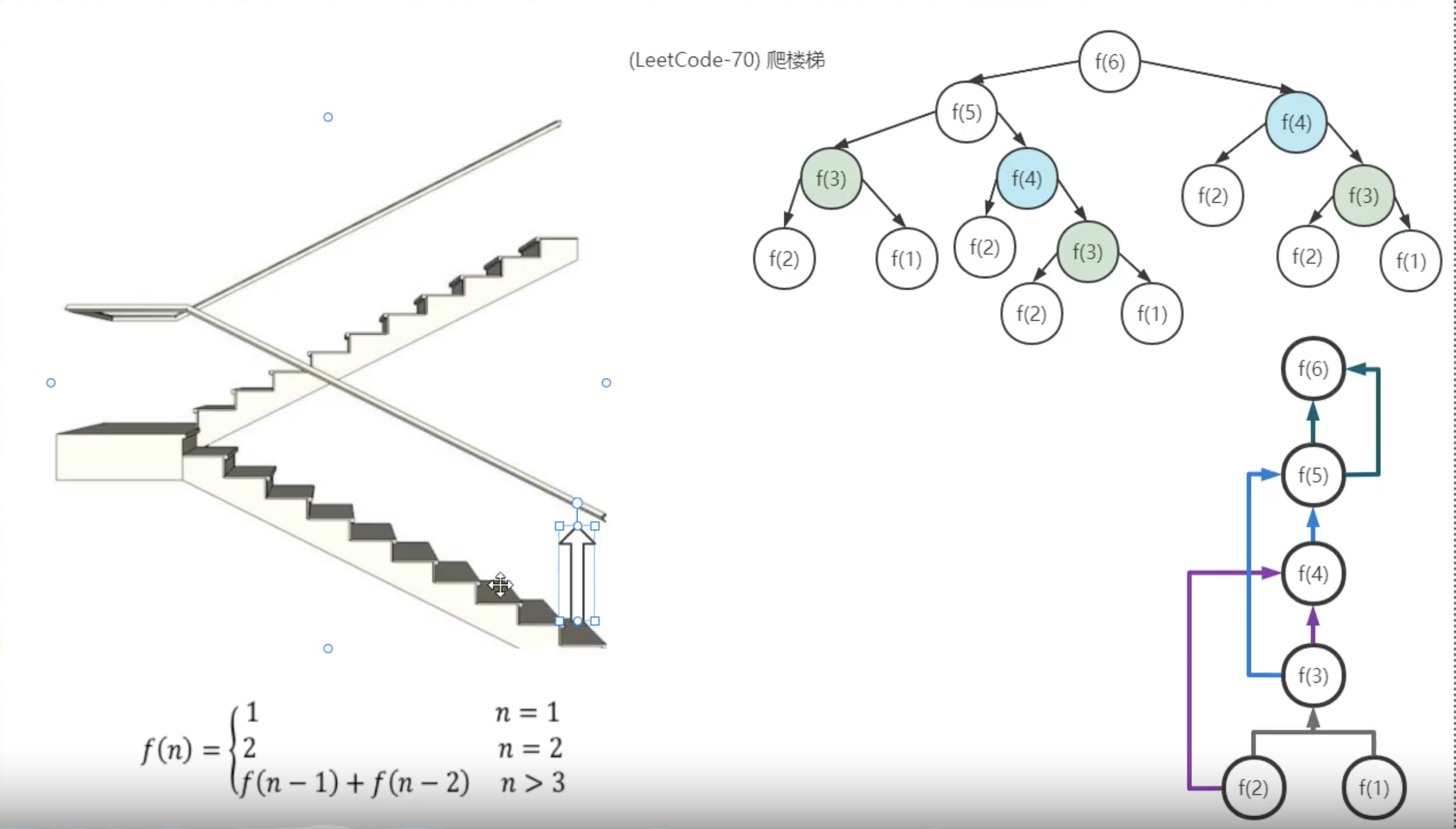﻿• 价格透明
• 信息保密
• 进度掌控
• 售后无忧# 算法思想-动态规划

## 爬楼梯

``````假设你正在爬楼梯。需要 n 阶你才能到达楼顶。

1. 1 阶 + 1 阶
2. 2 阶

1. 1 阶 + 1 阶 + 1 阶
2. 1 阶 + 2 阶
3. 2 阶 + 1 阶
``````### 递归解决

``````class Solution {
public int climbStairs(int n) {
if (n==1){
return 1;
}else if (n==2){
return 2;
}else {
return climbStairs(n-1)+climbStairs(n-2);
}
}
}
``````

### 记忆化递归

``````class Solution {
public int climbStairs(int n) {
if (n==1){
return 1;
}
if (n==2){
return 2;
}
Map<Integer,Integer> map=new HashMap<>();
if (null!=map.get(n)){
return map.get(n);
}else {
int result= climbStairs(n-1)+climbStairs(n-2);
map.put(n,result);
return result;
}
}
}
``````

### 动态规划

``````class Solution {
public int climbStairs(int n) {
if (n==1){
return 1;
}
if (n==2){
return 2;
}
int[] limit = new int[n+1];
limit=1;
limit=2;
//新建一个数组，里面的limit是空的，其余的从1开始的，也就是n，对应的爬楼梯的方法的和，就是3楼有3种方法，4楼有5种方法。
//这就是为什么长度是n+1，因为第0个是空的
for (int i=3;i<=n;i++){
limit[i]=limit[i-1]+limit[i-2];
}
return limit[n];
}
}
``````### 低价透明### 金牌服务### 信息保密